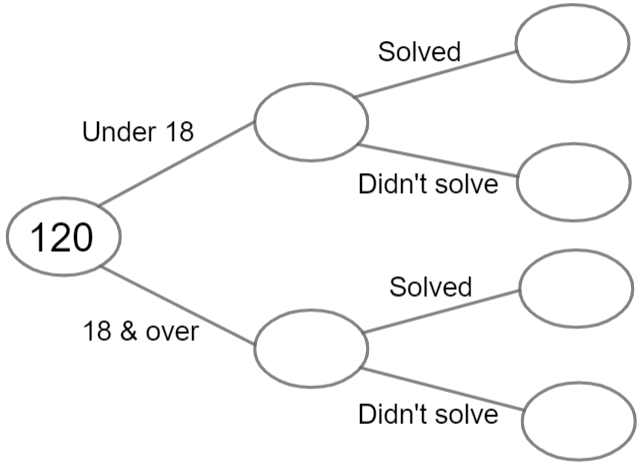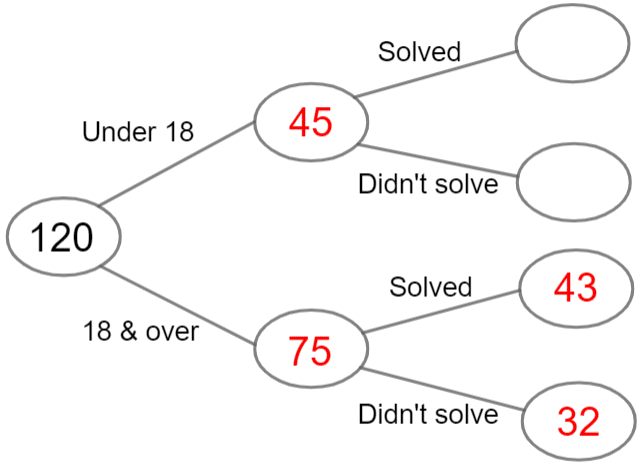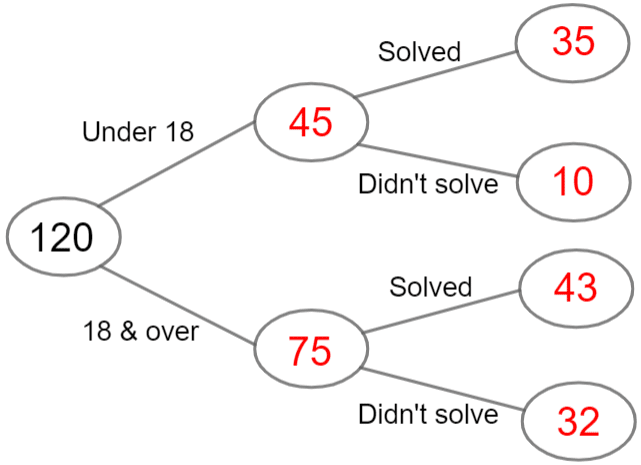# Frequency Trees

GCSELevel 4-5AQAEdexcelOCR

## Frequency Trees

A frequency tree is used to show how a group of people/things can be broken up into certain categories.

Make sure you are happy with the following topics before continuing.

Level 4-5GCSEAQAEdexcelOCR

## Constructing Frequency Trees

Data from experiments that have $2$ or more steps can be recorded using a frequency tree. For example:$120$ people were given $3$ minutes to solve a puzzle.

• $45$ people who tried to solve the puzzle were under $18$ years old.
• $78$ people solved the puzzle.
• $32$ people aged $18$ and over did not solve the puzzle.

Complete the frequency tree using this information.

Step 1: $\textcolor{red}{45}$ of the people in question were under $18$.

The total number of people is $120$. We can now work out the following.

$\text{‘18 and over’ people }=120-45=\textcolor{red}{75}$We can now add these to the frequency tree.

Step 2:$\textcolor{red}{32}$ people aged $18$ & over did not solve the puzzle’.

We know $\textcolor{red}{32}$ people aged $18$ and over did not solve the puzzle, so this can be added to the frequency diagram.

Next, we calculate how many people aged $18$ and over solved the puzzle.

$\text{‘18 and over’ and ‘solved puzzle’ }=75-32=\textcolor{red}{43}$

Step 3: For the final two blank spaces, we can’t simply use the same technique as before, we have to look at the second bit of information:

We know ‘$78$ people solved the puzzle’We now know how many of the ‘$18$ and over’ group solved the puzzle, so

$\text{‘under 18’ and ‘solved puzzle’ }=78-43=\textcolor{red}{35}$

Lastly, this number and the ‘under $18$ – didn’t solve’ group must add up to $45$, so we get

$\text{‘under 18’ and ‘didn’t solve puzzle’}=45-35=\textcolor{red}{10}$

Level 4-5GCSEAQAEdexcelOCR

## Using Frequency Trees

We can now use the frequency trees we have created to answer probability questions.

Example: Using the frequency tree from the previous example, if you choose one of the under $18$s at random, what is the probability that they did not solve the puzzle?

From our diagram we can see that there are $45$ under $18$s, $10$ of which didn’t solve the puzzle. Therefore, the probability is

$\dfrac{10}{45}=\dfrac{2}{9}$

This fraction is now in its simplest form, so we’re done.

Level 4-5GCSEAQAEdexcelOCR

## Frequency Trees Example Questions

Since there were $200$ people in total, of which $58$ travelled by train, we can calculate how many travelled by bus:

$200-58=142 \text{ travelled by bus }$

We are also told that of the total number of people who travelled by bus – which we now know is $142$ people – $40$ were late. If we know how many were late, we can easily work out how many who travelled by bus were not late:

$142-40=102 \text{ by bus and on time}$

So, that leaves two remaining bubbles in the tree diagram, the ones branching off from the $58$ people who took the train.

The question tells us that $71$ people in total were late to the event. Given that $40$ of those people who were late took the bus, we can calculate how many of these people who were late took the train:

$71-40=31\text{ late by train}$

Finally, if $31$ of the $58$ people who took the train were late, we can calculate how many took the train and were on time

$58-31=27\text{ by train and on time}$

The completed frequency tree should therefore look like this:Gold Standard Education

a) First of all, if there are $75$ people in total and $63$ are right-handed, we can perform a simple calculation to work out how many people are left-handed:

$75-63=12$ left-handed people

Secondly, we know that of the left-handed people, one third are right-footed. So the number of people who are left-handed and right-footed can be calculated as follows:

$12\div 3=4$ left-handed and right-footed people

Furthermore, since there are $12$ left-handed people in total, we can now work out how many of them are left-footed:

$12-4=8$ left-handed and left-footed people

So, we just have two remaining bubbles, the ones branching off from the $63$ right-handed people.

The final piece of information given to us in the question tells us that $27$ people in total are left-footed. Given that we now know that $8$ of the left-handed people are left-footed, we can calculate the number of people who are right-handed and left-footed:

$27-8=19$ right-handed and left-footed people

Finally, if $19$ of the $63$ right-handed people are left-footed, we can calculate how many are right-handed and right-footed

$63-19=44$ right-handed and right-footed people

The completed frequency tree should therefore look like this:b) There are $63$ people who are right-handed, and $44$ of them are right-footed. So, the probability of picking a right-handed person who is also right-footed is

$\dfrac{44}{63}$

c) There are $75$ people in total. The number of right-footed people is:

$44+4=48$

Therefore, the probability of selecting a right-footed person is:

$\dfrac{48}{75}$

This fraction can be simplified to:

$\dfrac{16}{25}$Gold Standard Education

a)  We have very little information to go on here, but since we know that $13$ students failed French and that this was $\frac{1}{12}$ of the entire French group, we can work out how many students took French:

$12 \times13 = 156\text{ French students in total}$

If $156$ students took French and $13$ failed, then the number that passed was:

$156-13=143\text{ took French and passed}$

We are also told that $65\%$ of the year group took French.  This represents $156$ students.  From this information, we can calculate the total number of students in the year group and then the number of students who took Spanish.

If

$65\% = 156\text{ students}$

then

$1\% = 156\div65\text{ students}$

so

$100\% = 156\div65\times100\text{ students}$

$156\div65\times100=240\text{ students}$

Therefore, there was a total of $240$ student in year $11$ that took French or Spanish.

Since $35\%$ of the year group took Spanish, the exact number that took Spanish can be calculated as follows:

$0.35 \times 240\text{ students} = 84\text{ Spanish students}$

We are told that the number of students who failed Spanish was twice the number that failed French.  Since $13$ failed French, we can work out the number that failed Spanish:

$2\times13 = 26\text{ failed Spanish}$

Therefore the number that passed Spanish can be calculated as follows:

$84-26=58\text{ passed Spanish}$

The completed frequency tree should therefore look like this:b)  We know that the total in the year group was $240$ students.  Of these $240$, $143$ passed French.  As a percentage, this can be calculated as follows:

$\dfrac{143}{240}\times100=60\%\text{ to the nearest whole number}$Gold Standard Education

a)  The first fact that we are presented with is that $15\%$ of the pupils chose a cheese sandwich on brown bread and that this figure represents a total of $18$ pupils.  From this information, we can work out the total number of pupils who had a cheese sandwich.

If

$15\% = 18\text{ pupils}$

then

$1\% = 18 \div15\text{ pupils}$

so

$100\% = 18 \div15 \times 100\text{ pupils}$

$18 \div15 \times 100 = 120\text{ pupils who chose cheese}$

If $120$ pupils chose a cheese sandwich, and $18$ of them had brown bread, then we can easily calculate the number that had a cheese sandwich on white bread:

$120-18 = 102\text{ cheese sandwich on white bread}$

The next key piece of information we are given is that the ratio of pupils who chose a cheese sandwich to pupils who chose a ham sandwich is $2 : 3$.  From this information we can deduce that $\frac{2}{5}$ of these pupils had a cheese sandwich and $\frac{3}{5}$ of this pupils had a ham sandwich.  (We are dealing in fifths here because the sum of the ratio is $5$.)

If

$\dfrac{2}{5} = 120\text{ pupils}$

then

$\dfrac{1}{5} = 60\text{ pupils}$

so

$\dfrac{3}{5} = 180\text{ pupils}$

Therefore we can conclude that a total of $180$ students chose a ham sandwich.

At this point we now also know that there was a total of $300$ pupils:

$120\text{ cheese} + 180\text{ ham} = 300\text{ pupils total}$

Finally, we know that of the people that chose a ham sandwich, $\frac{3}{10}$ opted for brown bread.  We can now calculate exactly how many pupils this is since we have now worked out the number of students who opted for the ham sandwich:

$\dfrac{3}{10}\times180 =54\text{ ham sandwich on brown bread}$

The number of students who had a ham sandwich on white bread is simply the total that chose a ham sandwich minus the $54$ who chose a ham sandwich on brown bread:

$180-54=126\text{ ham sandwich on white bread}$

The completed frequency tree should therefore look like this:b)  We know from the completed frequency tree that there was a total of $300$ pupils and that a total of $52$ pupils chose a ham sandwich on brown bread.  The probability of selecting a pupil at random from the group who chose a ham sandwich on brown bread can be expressed as follows:

$\dfrac{54}{300}$

This can be simplified to:

$\dfrac{27}{150}$

This can again be simplified to:

$\dfrac{9}{50}$Gold Standard Education

In this question, we have a couple of ratios and some percentages, but the only precise figure we have to start with is the total number of people who voted for the Green Party.

We know that the votes were shared between the $3$ parties in the ratio of $7 : 6 : 3$.  This means that the Conservative Party received $\frac{7}{16}$ of the votes, the Labour party $\frac{6}{16}$ and the Green Party $\frac{3}{16}$.  (We are dealing in sixteenths here because the sum of the ratio is $16$.)

If $24,750$ voted for the Green party and this represented $\frac{3}{16}$ of the total number of votes received, then we can work out the total number of votes:

If

$\dfrac{3}{16} = 24,750\text{ votes}$

then

$\dfrac{1}{16} = 24,750\div3\text{ votes}$

so

$\dfrac{16}{16} = (24,750\div3)\times 16\text{ votes}$

$(24,750\div3)\times 16=132,000\text{ votes}$

So, if there was a total of $132,000$ votes, we can work out the number of votes received by the Conservative party and the Labour party.

$\dfrac{7}{16}\times132,000=57,750\text{ votes}$

$\dfrac{6}{16}\times132,000=49,500\text{ votes}$

We know that votes cast by men and by women for the Labour party were in a ratio of $3 : 2$.  This means that $\frac{3}{5}$ of the votes were cast by men and $\frac{2}{5}$ by women.  (We are dealing in fifths here because the sum of the ratio is $5$.)

Since we know the total number of votes received by the Labour party and the fraction cast by men and women, we can work out the exact number of votes made by each gender.

The total number of votes cast by men was:

$\dfrac{3}{5}\times49,500=29,700\text{ votes}$

The total number of votes cast by women was:

$\dfrac{2}{5}\times49,500=19,800\text{ votes}$

We are also told that the number of female votes received by the Green party was $65\%$ of the total number of female votes received by the Labour party.  Since the total number of female votes received by the Labour party was $19,800$, we simply need to work out $65\%$ of this amount:

$0.65\times19,800 = 12,870\text{ votes}$

Since the Green party received $24,750$ votes in total, we can work out the number of male votes:

$24,750-12,870=11,880\text{ male votes}$

The final thing to work out is the number of male and female votes received by the Conservative Party.  We know that the number of male votes was $40\%$ more than the total of male votes received by the Labour party.  The total number of male votes received by the Labour party was $29,700$, so we will need to increase this figure by $40\%$, as follows:

$29,700\times1.4 = 41,580\text{ male votes}$

If the Conservative party received $57,750$ votes in total, of which $41,580$ were male, then the number of female votes can be calculated as follows:

$57,750-41,580=16,170\text{ female votes}$

The completed frequency tree should, therefore, look like this:Gold Standard Education

## Frequency Trees Worksheet and Example Questions

### (NEW) Frequency Trees Revision Exam Style Questions - MME

Level 1-3GCSENewOfficial MMEGold Standard Education

£19.99 /month. Cancel anytime

Level 1-3GCSE